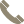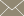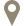Home » Faculty Profiles » Faculty

Assistant Professor

#### CONTACT INFO(956) 326-2594m.mohebujjaman@tamiu.eduLBV-385B

#### COURSES TAUGHT

• Calculus II (MATH 2414)
• Calculus III (MATH 2415)
• College Algebra (MATH 1314)
• Discrete Mathematics (MATH 3365)
• Fundamentals of Mathematics I (MATH 1350)
• Graduate Research in Math (MATH 5395)
• Numerical Linear Algebra (MATH 4330)
• Partial Differential Equations (MATH 4350)
• Pre-Calculus (MATH 2412)
• Real Analysis I (MATH 5305)
• Statistical Analysis (MATH 3360)
• Thesis I (MATH 5398)
• Undergraduate Research in Math (MATH 4380)